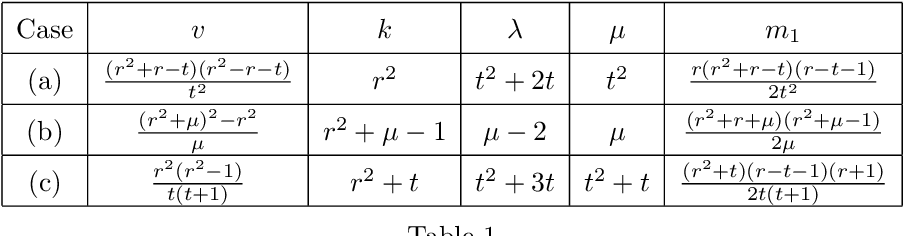Skip to search formSkip to main content
You are currently offline. Some features of the site may not work correctly.

# Conference graph

In the mathematical area of graph theory, a conference graph is a strongly regular graph with parameters v, k = (v − 1)/2, λ = (v − 5)/4, and μ = (v… Expand
Wikipedia

## Papers overview

Semantic Scholar uses AI to extract papers important to this topic.
2018
2018
Abstract Suppose there exists a Hadamard 2- ( m , m − 1 2 , m − 3 4 ) design having skew incidence matrix. If there exists a… Expand
2018
2018
• Math. Comput. Sci.
• 2018
• Corpus ID: 53116120
Suppose there exists a Hadamard 2-$$(m,\frac{m-1}{2},\frac{m-3}{4})$$(m,m-12,m-34) design with skew incidence matrix, and a… Expand
Review
2014
Review
2014
• 2014
• Corpus ID: 32370755
Given a graph G; let kGk denote the trace norm of its adjacency matrix, also known as the energy of G: The main result of this… Expand
2010
2010
• WAIM
• 2010
• Corpus ID: 46564151
Information Extraction (IE) aims at mining knowledge from unstructured data. Terminology extraction is one of crucial subtasks in… Expand
2010
2010
We say that a regular graph G of order n and degree r>=1 (which is not the complete graph) is strongly regular if there exist non… Expand
2009
2009
• SDM
• 2009
• Corpus ID: 17521132
Given an author-paper-conference graph, how can we automatically find groups for author, paper and conference respectively… Expand
•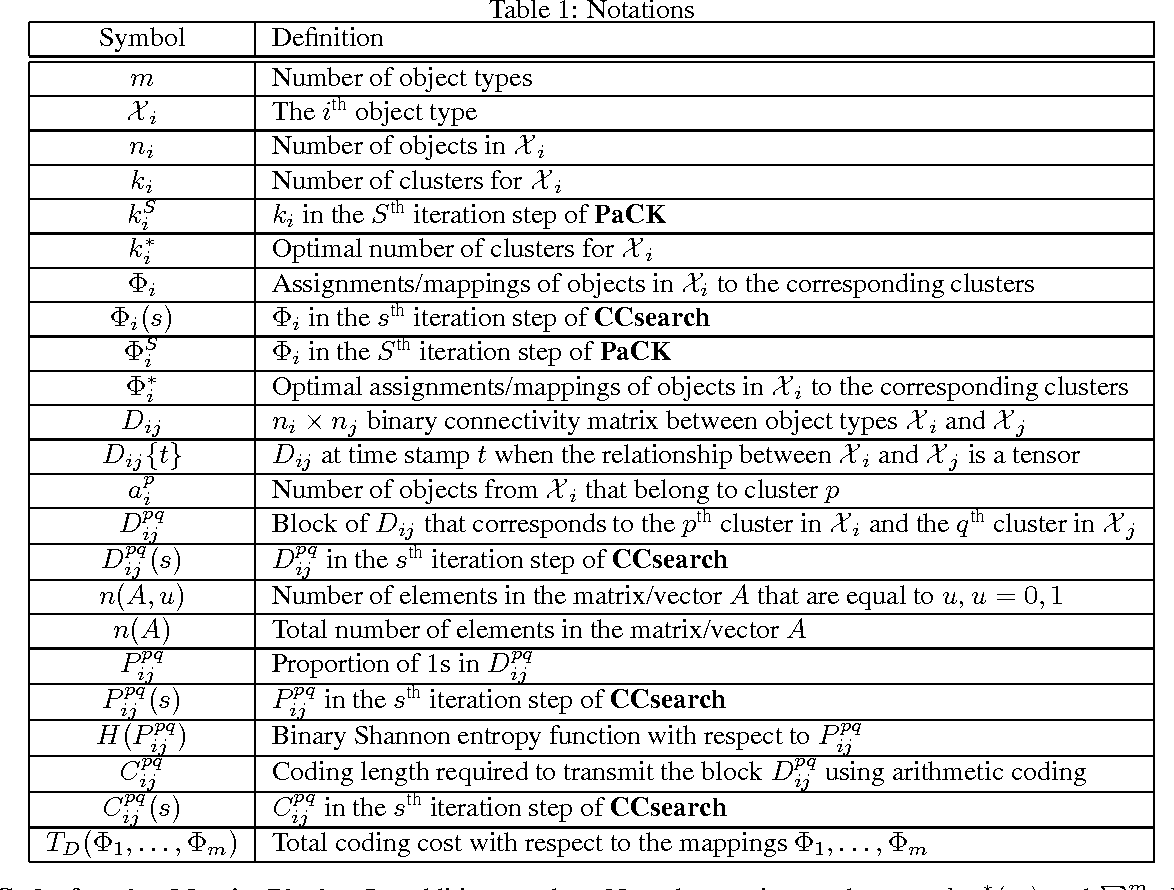•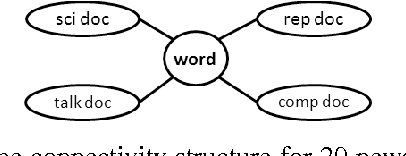•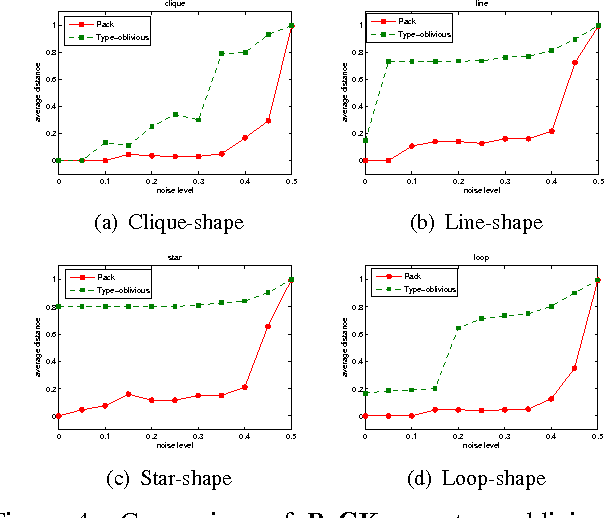•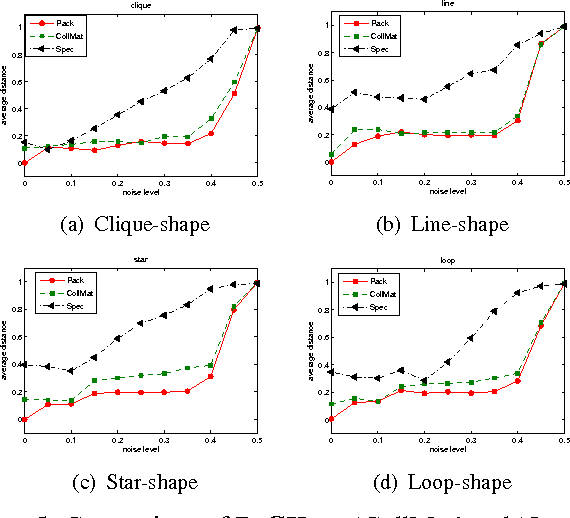•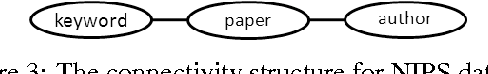2009
2009
• CIKM
• 2009
• Corpus ID: 15779175
Given an author-conference graph, how do we answer proximity queries (e.g., what are the most related conferences for John Smith… Expand
•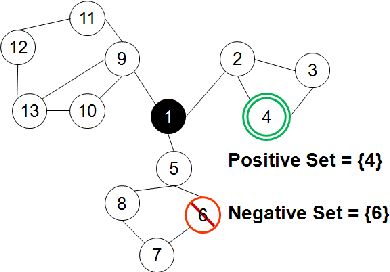•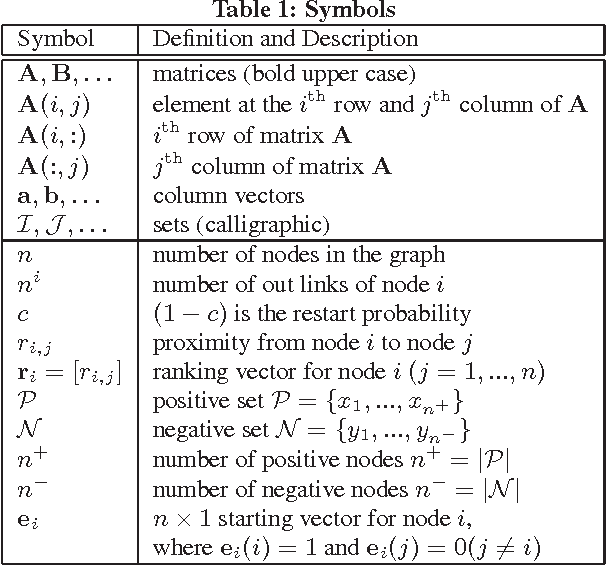•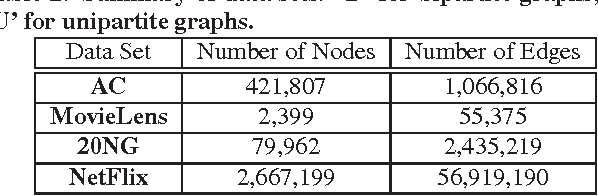•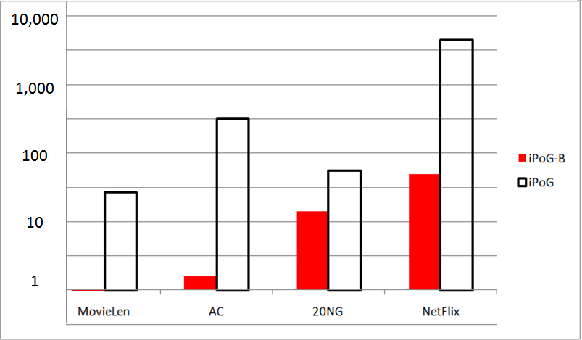2008
2008
• APWeb
• 2008
• Corpus ID: 1441153
Recent models have introduced the notion of dimensions and hierarchies in social networks. These models motivate the mining of… Expand
•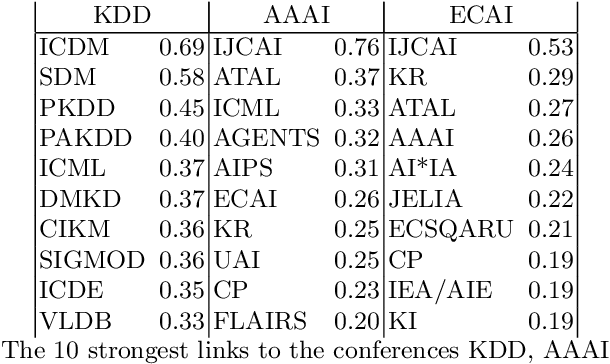•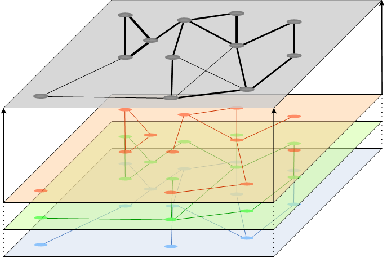•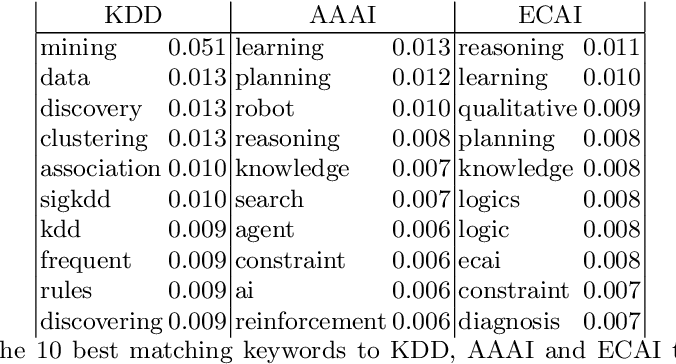•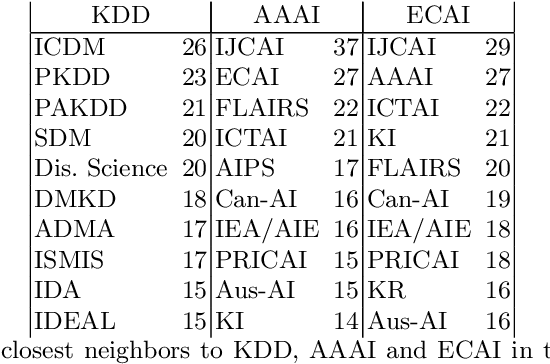•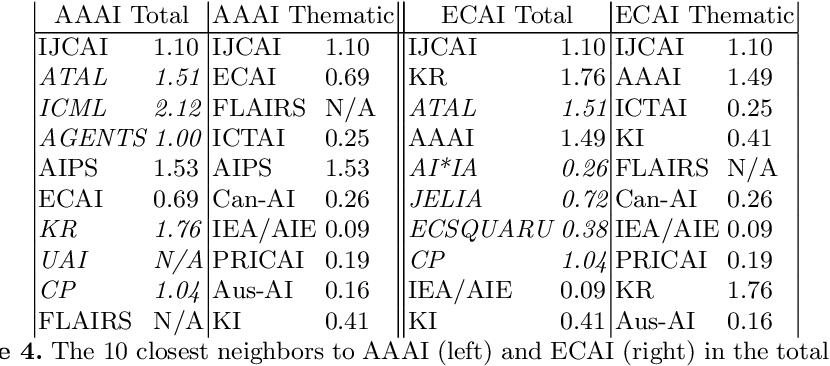2004
2004
• 2004
• Corpus ID: 34709986
We determine the Nomura algebras of the type-II matrices belonging to the Bose-Mesner algebra of a conference graph.
2003
2003
• 2003
• Corpus ID: 18836243
Given λ and µ, it is shown that, unless one of the relations (λ − µ)2 =4 µ, λ − µ = −2, (λ − µ) 2 +2( λ − µ )=4 µ is satisfied… Expand
•